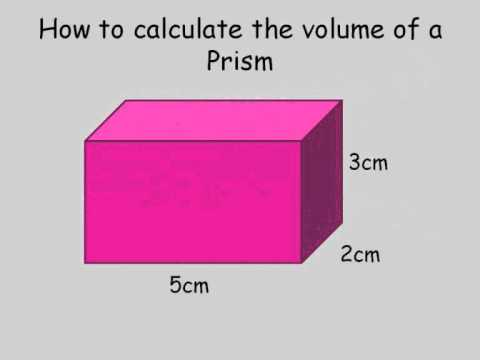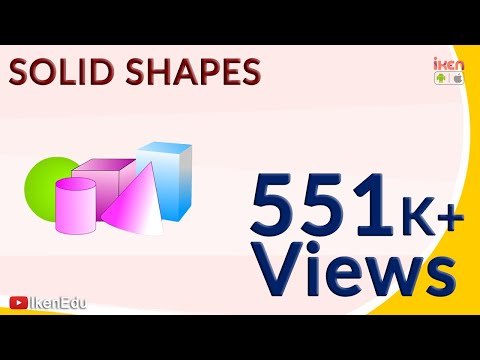## Solids

Subject: Compulsory Maths

Find Your Query

#### Overview

Solid means having three dimensions (length, breadth, and thickness), as a geometrical body or figure. Or it may also defined as relating to bodies or figures of three dimensions.

##### Solids

Solid means having three dimensions (length, breadth and thickness), as a geometrical body or figure. Or it may also be defined as relating to bodies or figures of three dimensions.

#### Prism and their surface area and volume

Prisms are the solid object that has two opposite faces congruent and parallel.The above figures are different solid figures which have congruent opposite face.

Each congruent face of a prism is called its cross-section. There are infinite numbers of imaginary surfaces that cut the prism perpendicular to its height or length with congruent surfaces.

Total surface area of prism

Let's take a cartoon box. While unfolding the cartoon box, there we can find many faces of the boxes. Measure all the surfaces and find the area of each surface. If the length is “l” breadth is “b” and height is ‘h’, what will be the total surface area?

In a box, altogether there are 6 surfaces. Among them three pairs are congruent. Thus, total surface area (A) = 2(l $\times$ b) + 2(b $\times$h) + 2(l $\times$ h) square units

= 2(lb + bh + lh) square units.

In the case of irregular shape, area of surface are calculated separately and added to find out the total surface area.

Lateral surface area of prism

The sum of areas of four lateral surfaces of the prism is called the lateral surface area of the prism.

Lateral surface area (S) = 2(l $\times$ h) + 2(b $\times$ h)

= 2lh + 2bh

= h $\times$ 2(l+b)

= h $\times$ p

$\therefore$ lateral surface area (S) = height $\times$ perimeter of base

Total surface area of the prism (TSA) = Lateral surface area + 2 $\times$ area of cross section

i.e. TSA =LSA + 2A

Volume of prism

let's take a cuboidal prism.

 For a cuboid For a cubeVolume (V) = l $\times$ b $\times$ h V = A $\times$ H Where, A = l $\times$ b Volume (V) = l $\times$ l $\times$ l (V) = l2 $\times$ l (V) = A $\times$ l

Thus, Volume = Area of cross-section × height

 S.N Solid Figures Area of base or cross section Lateral Surface Area Total surface Area Volume 1. Cuboid A = l $\times$ b 2h(l + B) 2(lb + bh + lh) V = l$\times$ b$\times$ h 2. Cube A = l2 4l2 or 4A 6l2or 6A V = l3 3. Prism A = base area or A = area of cross section h $\times$ p p$\times$ h + 2A V = A$\times$ h
##### Things to remember
• Lateral Surface Area (LSA) = height $\times$ perimeter of base
• Total Surface Area of the prism (TSA) = Lateral surface area + 2 $\times$ area of cross section
• Volume of cuboid (V) = l $\times$ b $\times$ h

V = A $\times$ H

Where, A = l $\times$ b

• Volume of cube(V) = l $\times$ l $\times$ l

(V) = l2 $\times$ l

(V) = A $\times$ l

• It includes every relationship which established among the people.
• There can be more than one community in a society. Community smaller than society.
• It is a network of social relationships which cannot see or touched.
• common interests and common objectives are not necessary for society.
##### Videos for Solids##### How to calculate the volume of a rectangular prism.wmv##### Questions and Answers

Solution:

Here, length (l) = 8cm

Total surface area (TSA) = 6l2

= 6× (8cm)2

= 6× 64cm2

= 384cm2

Again,

Volume (V) = l3

= (8cm)3

= 512cm3

Hence, TSA = 384cm2 and volume = 512cm3

Solution:

Here,

Lateral Surface Area (LSA) = perimeter× height

= (1cm +cm + 4cm + 1cm + 8cm + 6cm + 8cm) × 6cm

= 34cm× 6cm

= 204cm2

Also,

Volume (V) = Area of cross section× height

= (8cm× 6cm - 4cm× 3cm)× 6cm

= (48cm2 - 12cm2)× 6cm

= 36cm2× 6cm

= 216cm3

Solution:

Here, taking only front section onlyArea of cross section (A) = (5× 4 + 9× 3 + 13× 3)cm2

= (20 + 27 + 39)cm2

= 86cm2

Lateral surface area (LSA) = p× l

= (5 + 4 + 4 + 3 + 4 + 3 + 13 + 10)cm× 3cm

= 46cm + 3cm

= 138cm2

Total surface area (TSA) = LAS + 2A

= 138cm2 + 172cm2

= 310cm2

Volume of the prism (V) = A× h

= 86cm2× 3cm

= 258cm3

Solution:

Here, taking front section of the figureArea of cross section (A) = 9cm× 15cm + 18cm × 15cm

= 135cm2 + 270cm2

= 405cm2

Lateral Surface Area (LSA) = p× l

= (30 + 18 + 9 + 15 + 9 + 15)cm × 10cm

= 96cm× 10cm

= 960cm2

Total Surface Area (TSA) = LSA + 2A

= 960cm2 + 2 × 405cm2

= 960cm2 + 810cm2

=1770cm2

Volume of the prism (V) = A × h

= 405cm2× 10cm

= 4050cm3

Solution:

Here,

Length (l) = 10cm

Breadth (b) = 5cm

Heigth (h) = 8cm

Now,

LSA = 2h(l + b)

= 2× 8cm (10cm + 5cm)

= 16cm× 15cm

= 240cm2

Again,

TSA = 2(lb + bh + lh)

= 2 (10cm× 5cm + 5cm× 8cm +10cm× 8cm)

= 2 (50cm2 + 40cm2 + 80cm2)

= 2× 170cm2

= 340cm2

Also,

Volume (V) = l× b× h

= 10cm× 5cm× 8cm

= 400cm3

Solution:

Here,

Length of box (l) = 30cm

Breadth of box (b) = 20cm

Height of box (h) = 10cm

Length of soap (l1) = 3cm

Breadth of soap (b1) = 2cm

Height of soap (h1) = 2cm

Now,

Volume of box (V) = l× b× h

= 30cm× 20cm× 10cm

= 6000cm3

Volume of soap (V1) =l1× b1× h1

= 3cm × 2cm ×2cm

= 12cm3

∴ Number of soap that can be fitted on the box (N) = $\frac{V}{V_1}$

= $\frac{600cm^3}{12cm^3}$

= 50

Hence, 50 soap can be fitted on the box.

Solution:

Here,

Length (l) = 16m

Breadth (b) = 15m

Heigth (h) = 13m

Area of 4 walls (A) = 2h(l + b)

= 2× 13m (16m + 15m)

= 26m× 31m

= 806m2

Cost of painting wall (C) = Rs 120m2

Total Cost (T) = ?

∴ Total Cost (T) = C× A

= Rs 120 ×806m2

= Rs 96,720

Solution:

Here,

Volume of each brick (V1) = l1 × b1 × h1

=15cm× 10cm× 5cm

= 750cm3

Volume of wall (V) = l × b × h

=20,000cm× 500cm× 30cm

= 300,000,000cm3

Now,

Number of Bricks (N) = $\frac{V}{V_1}$

= $\frac{300,000,000cm^3}{750cm^3}$

= 400,000

Again,

The cost of 1,000 bricks = Rs 17,000

Cost of 1 bricks = $\frac{Rs 17,000}{1,000}$

Cost of 400,000 bricks = $\frac{Rs 17,000}{1,000}$× 400,000 = Rs 6,800,000

∴ Rs 6,800,000 is required to make the wall.

 LSA TSA The lateral surface area of a three-dimensional object is the surface area of the object minus the area of its bases. The total surface area of a three- dimensional object is the surface area of the object adding all of its areas. For example, dice. We found that the surface area of a six-sided dice.Since the dice has two bases, we subtractthe area of the two bases. For example,dice. We found that the surface area of a six-sided dice. We add all the areas of a dice.

Solution:

Here,

Length of wall (l) = 300m = 30000cm

Breadth of wall (b) = 1m = 100cm

Height of wall (h) = 4.5m = 450cm

Length of brick (l1) = 300m = 30000cm

Breadth of brick (b1)= 1m = 100cm

Height of brick (h1) = 4.5m = 450cm

Cost of brick = Rs 13

Cost of Transport = Rs 1.20

Cost of load/unload = Rs 0.20

Total cost of a brick = Rs (13 + 1.20 + 0.20) = Rs 14.4

Now,

Volume of a brick (V1) =20cm× 10cm× 5cm

= 1000cm3

Volume of wall (V) = 30000cm × 100cm× 450cm

= 1,350,000,000cm3

∴ Number of bricks (N) = $\frac{V}{V_1}$

= $\frac{1,350,000,000cm^3}{1000cm^3}$

= 1,350,000

Cost of 1 brick = Rs 14.4

Cost of1,350,000 = Rs 14.4× 1,350,000 = Rs 19,440,000

∴ Total cost of constructing a wall is Rs 19,440,000.

Solution:

Here,

Length of store (l) = 30m = 3000cm

Breadth of store (b) = 8m = 800cm

Heigth of store (h) = 6m = 600cm

Length of soap (l1) = 80cm

Breadth of soap (b1) = 60cm

Heigthof soap (h1) = 50cm

Now,

Volume of a store room (V) = l× b× h

= 3000cm× 800cm× 600cm

= 1,440,000,000cm3

Volume of packets of soap (v1) = l1× b1× h1

=80cm× 60cm× 50cm

= 2,40,000cm3

Then,

Number of soap of packets that can be fulfill the store room (N) = $\frac{V}{V_1}$

=$\frac{1,440,000,000cm^3}{2,40,000cm^3}$

= 6000

∴ 6000 soap of packets that can be fulfill the store room.

A solid object having opposites faces congruent and parallel is known as a prism. Cube and Square prism are the examples of a prism.

Solution:

Volume of a cubical tank (V) = 125m3

Total Surface Area (TSA) = ?

Now,

V = l3

Or, 125m3 = l3

or, l = (125m3)$\frac{1}{3}$

∴ l = 5m

Now, TSA = 6l2

= 6× (5m)2

= 150m2

Solution:

Length of tank (l) = 6m

Breadth of tank (b) = 4m

Height of tank (h) = 5m

Now,

Volume of a tank (V) = l× b× h

= 6m× 4m × 5m

= 120m3

Hence, the tank hold 120m3 = 120× 1000 = 120,000 litre of water.

Solution:

Perimeter (P) = 24cm

Height (h) = 5cm

Now,

Lateral surface area (LSA) = P× h

= 24cm × 5cm

= 120cm2

© 2019-20 Kullabs. All Rights Reserved.